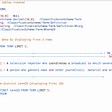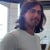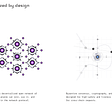# SMTChecker toward completeness

• loops are handled in a bounded way (only one iteration is considered);
• functions are analyzed separately in their own contexts.
`constructor(...);while(true)    random_public_function(...);`
`pragma experimental SMTChecker;contract C {    uint sum;    uint count;    function () external payable {        require(msg.value > 0);        // Avoid overflow.        require(sum + msg.value >= sum);        require(count + 1 > count);        sum += msg.value;        ++count;    }    function average() public returns (uint) {        require(count > 0);        uint avg = sum / count;        assert(avg > 0);        return avg;    }}`
`(and      (function_average_68_0 2 1 0 0)     (interface_C_69_0 2 1)     (fallback_42_0 0 0 2)     (interface_C_69_0 0 0))`
• `(interface_C_69 <sum> <count>)`is the contract’s idle state and the following numbers are the current values of state variables `sum` and `count`, respectively.
• `(fallback_42_0 <sum> <count> <msg.value>)` is a call to the fallback function.
• `(function_average_60_8 <sum> <count> <return_value> <avg>)` is a call to function `average`.
`function f(uint x) public pure {    uint y;    require(x > 0);    while (y < x)        ++y;    assert(y == x);}`
`function f() public pure {    uint x = 10;    uint y;    while (y < x)    {        ++y;        x = 0;        while (x < 10)            ++x;        assert(x == 10);    }    assert(y == x);}`
`uint[] a;function f(uint n) public {    uint i;    while (i < n)    {        a[i] = i;        ++i;    }    require(n > 1);    assert(a[n-1] > a[n-2]);}`
`function max(uint n, uint[] memory _a) public pure returns (uint) {    require(n > 0);    uint i;    uint m;    while (i < n) {        if (_a[i] > m)            m = _a[i];        ++i;    }    i = 0;    while (i < n) {        assert(m >= _a[i]);        ++i;    }    return m;}`
`pragma experimental SMTChecker;contract SimpleCrowdsale {   enum State { INIT, STARTED, FINISHED }   State state = State.INIT;   uint weiRaised;   uint cap;   function setCap(uint _cap) public {       require(state == State.INIT);       require(_cap > 0);       cap = _cap;       state = State.STARTED;   }   function () external payable {       require(state == State.STARTED);       require(msg.value > 0);       uint newWeiRaised = weiRaised + msg.value;       // Avoid overflow.       require(newWeiRaised > weiRaised);       // Would need to return the difference to the sender or revert.       if (newWeiRaised > cap)           newWeiRaised = cap;       weiRaised = newWeiRaised;   }   function finalize() public {       require(state == State.STARTED);       assert(weiRaised <= cap);       state = State.FINISHED;   }}`
`(and     (function_finalize_107_0 1 1 1)     (interface_SimpleCrowdsale_108_0 1 1 1)     (fallback_85_0 1 0 1 0)     (interface_SimpleCrowdsale_108_0 1 0 1)     (function_setCap_42_0 0 0 0 1)     (interface_SimpleCrowdsale_108_0 0 0 0))`

--

--

--

## More from Leonardo Alt

Building Solidity and Formal Verification tools.

Love podcasts or audiobooks? Learn on the go with our new app.

## Converting XML to Hive## How Kubernetes is used in industries and what all use cases are solved by Kubernetes?## X21 Digital & UnoRe Partnership## CS371p Spring 2022: Manasi Ramadurgum — Week 8## Bluechip-weekly Update #4 (2021/9/11~9/17)## Android: Optimizing Apps in your hands? — Part 1## Leonardo Alt

Building Solidity and Formal Verification tools.

## Custom Super Tokens## Axelar is a universal interoperability platform that connects all blockchains through a…## Announcing the Superfluid Developer SDK Suite## Build your First DAO and deploy it to moonbeam network PART 3 : Deploy to moonbeam.